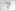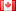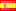Coroutine.sleep((1+ Math.random()))

Forum » Windower » Support » Coroutine.sleep((1+ math.random()))
Coroutine.sleep((1+ math.random()))DaneBloodOffline
Posts: 529
By DaneBlood 2018-10-30 15:38:48
so im not grat a lua so im mostly burchering stuff together

I stole the sleep/delay command from antnother plug
coroutine.sleep((1+ math.random()))

For me it reas like a delay os 1 sec + some undefine random delay.
for me the random delays is the important part. but where do i set the range of that random delay ?

from other languages i would assume something along math.rand(value) but is that correct ?

im wanting a random delay betwee 0 to 1 secsDaneBloodOffline
Posts: 529
By DaneBlood 2018-10-30 15:40:40
Bag sometimes i really needto give google a short try first

math.random ([m [, n]])

This function is an interface to the simple pseudo-random generator function rand provided by ANSI C. (No guarantees can be given for its statistical properties.)

When called without arguments, returns a uniform pseudo-random real number in the range [0,1). When called with an integer number m, math.random returns a uniform pseudo-random integer in the range [1, m]. When called with two integer numbers m and n, math.random returns a uniform pseudo-random integer in the range [m, n].

it just seems that its deafults to 0-1 range with real values when no value range is giving
perfect

However to get a Real value i need to use real number as input.
is just using 1.0 good enough to activate real number as output ?Quetzalcoatl.LanglyOffline
Server: Quetzalcoatl
Game: FFXI
user: Langly
Posts: 571
By Quetzalcoatl.Langly 2018-10-30 16:19:46
I don't think it's going to generate an imaginary number.DaneBloodOffline
Posts: 529
By DaneBlood 2018-10-30 16:47:37
Quetzalcoatl.Langly said: »
I don't think it's going to generate an imaginary number.
Kinda pointless comment as noone mentioned imaginary numbers.

I assume you are trying to make a cheap shot on real number and misunderstanding the context where the different is between real and integer number?

I give you a single HA for that good sir.

Its probably not going to give me ordinal numbers either (unless restricted withing natural numbers)Quetzalcoatl.LanglyOffline
Server: Quetzalcoatl
Game: FFXI
user: Langly
Posts: 571
By Quetzalcoatl.Langly 2018-10-30 17:16:15
Code
```math.random() generates pseudo-random numbers uniformly distributed. Supplying argument alters its behaviour:
math.random() with no arguments generates a real number between 0 and 1.
math.random(upper) generates integer numbers between 1 and upper (both inclusive).
math.random(lower, upper) generates integer numbers between lower and upper (both inclusive).
> = math.random()
0.0012512588885159
> = math.random()
0.56358531449324
> = math.random(100)
20
> = math.random(100)
81
> = math.random(70,80)
76
> = math.random(70,80)
75
upper and lower must be integer. In other case Lua casts upper into an integer, sometimes giving math.floor(upper) and others math.ceil(upper), with unexpected results (the same for lower).```DaneBloodOffline
Posts: 529
By DaneBlood 2018-10-30 17:44:11
Quetzalcoatl.Langly said: »
Code
```math.random() generates pseudo-random numbers uniformly distributed. Supplying argument alters its behaviour:
math.random() with no arguments generates a real number between 0 and 1.
math.random(upper) generates integer numbers between 1 and upper (both inclusive).
math.random(lower, upper) generates integer numbers between lower and upper (both inclusive).
> = math.random()
0.0012512588885159
> = math.random()
0.56358531449324
> = math.random(100)
20
> = math.random(100)
81
> = math.random(70,80)
76
> = math.random(70,80)
75
upper and lower must be integer. In other case Lua casts upper into an integer, sometimes giving math.floor(upper) and others math.ceil(upper), with unexpected results (the same for lower).```

Thank you but this doesn't answer my questions.

I'm asking if an input of 1.0 is going to be taking as an integer and thereby output integer numbers (like in your example list) or if the function is realize its a real number and output a real number as well ( just like when there is no input for the function)

Examples
math.random(1.0,2.0)
is this only going to give me either 1 or 2 (aka intergers)
or does it realize those are real numbers and give me e.g 1.7?

I' trying to establish if I needs to add in a tiny fraction to force it to go to real numbers of it will understand from the simple presence of the dot.

It will only give real numbers when no input is presentDaneBloodOffline
Posts: 529
By DaneBlood 2018-10-30 17:51:51
so to have a delay of 0 to 2 secs with a fluid range i would need to do

coroutine.sleep(math.random(0,20)/10)
?Bismarck.XurionOffline
Server: Bismarck
Game: FFXI
user: Xurion
Posts: 227
By Bismarck.Xurion 2018-11-01 07:10:28
Random number generation is never really random. In order to seed the "random" number generation, you should using something like this first:
Code
`math.randomseed(os.clock())`

The math.random function allows you to set up to two arguments; a minimum and a maximum value:
Code
```math.random() --> 0.30195013275552 (for example)
math.random(5)  --> a number from 1 to 5
math.random(5, 10)  --> a number from 5 to 10
```

Your sleep function above would produce one of the following:

Random 20 / 10 = 2
Random 19 / 10 = 1.9
...
Random 1 / 10 = 0.1
Random 0 / 10 = 0Offline
Posts:
By 2018-11-01 08:45:53
Post deleted by User.Bismarck.XurionOffline
Server: Bismarck
Game: FFXI
user: Xurion
Posts: 227
By Bismarck.Xurion 2018-11-02 16:21:32
coroutine.sleep(delay) is a delay in seconds that prevents the rest of the function/procedure from executing.

math.random() will return the values I mentioned in my last post. If you wanted it to return a number between 1 and 3, you need to called math.random(1, 3).DaneBloodOffline
Posts: 529
By DaneBlood 2018-11-07 17:09:30
Bismarck.Xurion said: »
coroutine.sleep(delay) is a delay in seconds that prevents the rest of the function/procedure from executing.

math.random() will return the values I mentioned in my last post. If you wanted it to return a number between 1 and 3, you need to called math.random(1, 3).

But i dont want and intergear results

math.random(1, 3) is going to give me only 3 diffrent results
1
2
3

I want to get 1.2 2.4 2.7 etcRagnarok.KenshiOffline
Server: Ragnarok
Game: FFXI
user: KenshiDRK
Posts: 64
By Ragnarok.Kenshi 2018-11-07 17:54:58
Code
```number = math.random(1, 3) + math.random()
coroutine.sleep(number)
```Bismarck.XurionOffline
Server: Bismarck
Game: FFXI
user: Xurion
Posts: 227
By Bismarck.Xurion 2018-11-09 03:43:13
When you don't provide math.random with arguments, it will give you a number between 0 and 1. For example, 0.84018771676347.

Multiply this by the max number you want - in your case, 3. There's no need to use math.random twice.
Code
```coroutine.sleep(math.random() * 3)
```